# Classifying triangles#### All in One Place

Everything you need for JC, LC, and college level maths and science classes.#### Learn with Ease

We’ve mastered the national curriculum so that you can revise with confidence.#### Instant Help

0/1
##### Intros
###### Lessons
1. Naming types of triangles based on their properties
1. By angles
• Acute
• Right
• Obtuse
2. By sides
• Scalene
• Isosceles
• Equilateral
0/11
##### Examples
###### Lessons
1. Classify the triangle by its sides and angles.

1.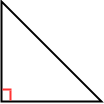2.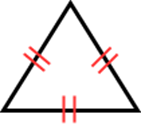3.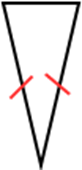4.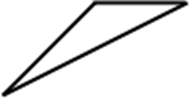2. Draw and classify the triangle by its vertices. Then, determine if it is a right triangle.
1. A(3, 6), B(3, 1), C(9, 1)
2. A(2, 4), B(5, 10), C(5, -2)
3. A(8, 7), B(11, 3), C(4, 2)
3. Find x. Then, classify the triangle by its angles.

1.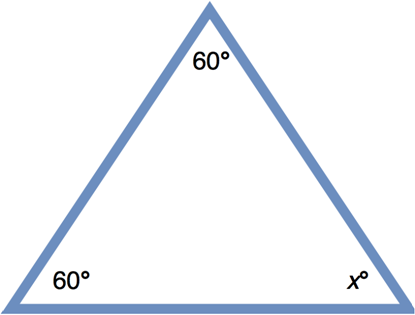2.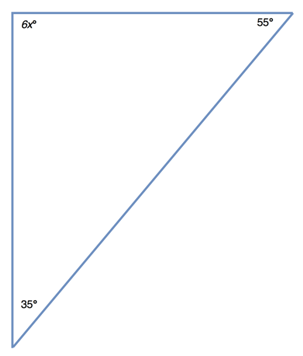3.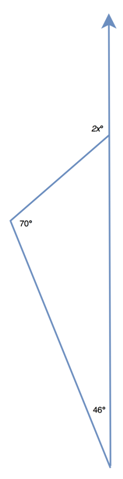4. One angle of a triangular chocolate box is 20°. The measure of the second angle is 3 times smaller than the third one. Find the measures of the two unknown angles.